# Rolling a Die

Rolling a Die

Most board games such as Monopoly, Snakes, and Ladders, and the likes involve the rolling of a die.

While playing these games, we hope for a certain number so that we can defeat our opponent, but the number that appears isn't always in our favor.

What is the probability that the number we are looking for is the one that will appear on the die?

Try out this interactive simulation to see if your luck favors you in the game of die.

## Lesson Plan

 1 What Do You Mean by Rolling a Die? 2 Important Notes on Rolling a Die 3 Solved Examples on Rolling a Die 4 Thinking Out of the Box! 5 Interactive Questions on Rolling a Die

## What Do You Mean by Rolling a Die?

As our random experiment, let us consider rolling a cubical die. This forms an event.

### Sample Space

The set of all the possible outcomes is called the sample space. Thus the numbers on the die become the set.

The sample space has six possible outcomes: {1, 2, 3, 4, 5, 6}.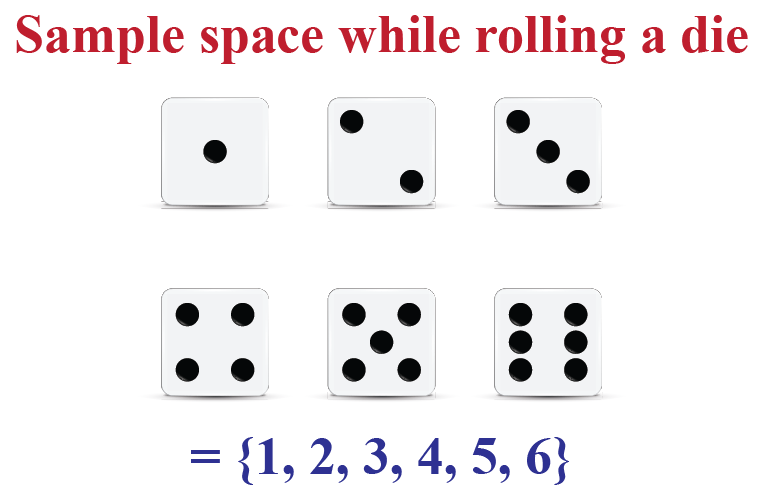### Fair Die

If the die has been constructed in a perfectly symmetrical manner, then we can expect that no outcome will be preferred over the other.

Thus, for a large number of rolls, the relative occurrence of each outcome should be roughly one-sixth $$\dfrac{1}{6}$$.

 \begin{align}P\left( F \right) = \frac{{{\rm{No}}{\rm{. of \,favorable\,\, outcomes}}}}{{{\rm{No}}{\rm{. of \,total\,\, outcomes}}}} = \frac{1}{6}\end{align}

Each of the 6 possible outcomes in a fair die has an even chance to appear.

The probability of getting any 1 number is $$\dfrac{1}{6}$$.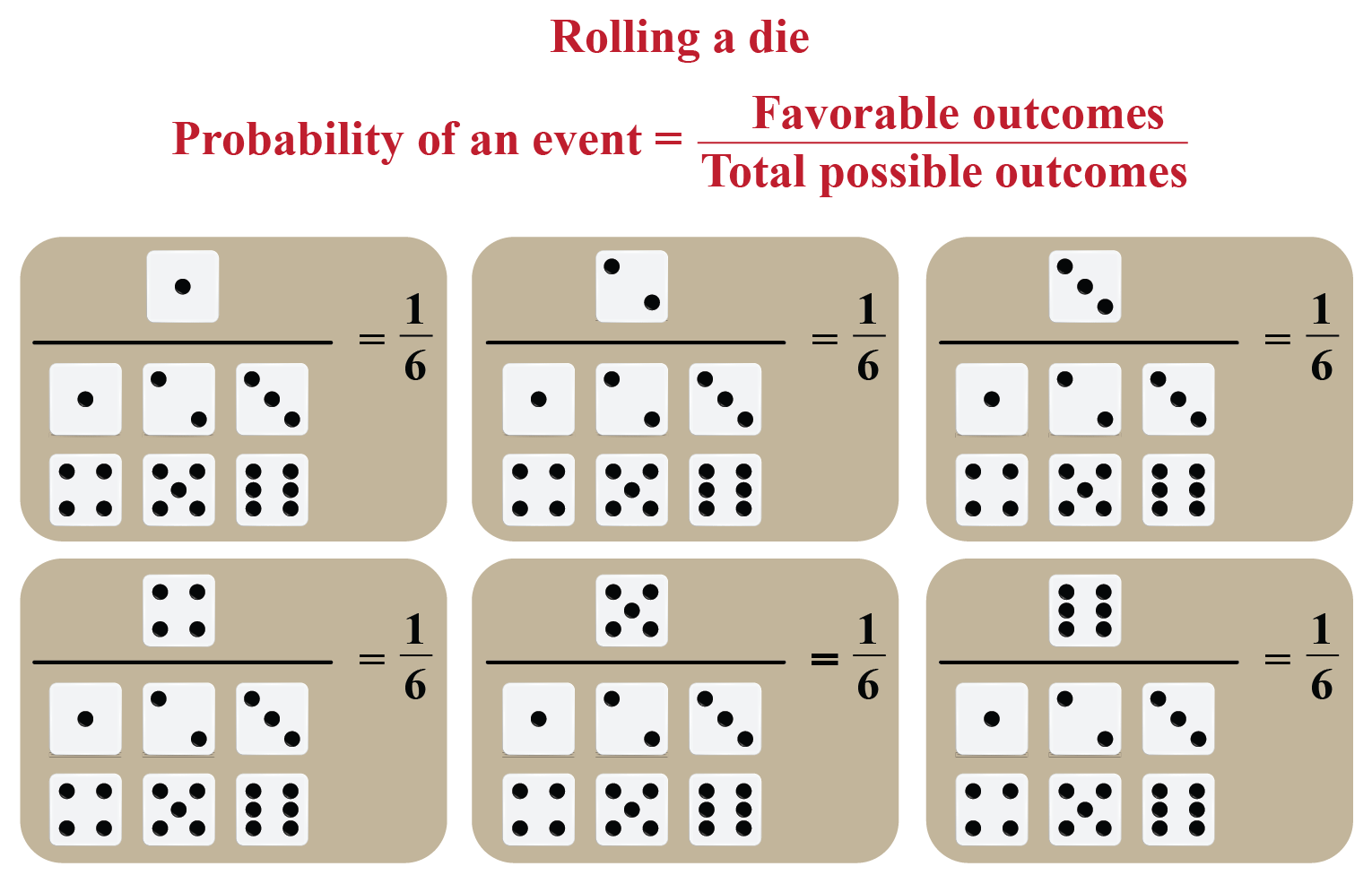We see that in the probability line below, the probability of an event is $$\dfrac{1}{6}$$ which lies in the least likely occurring zone. When 0 indicates impossibility, 1 indicates the event certain to happen. Rolling a die has the possibility of 0, which is more unlikely to happen. This is because the sample space has 6 possible outcomes.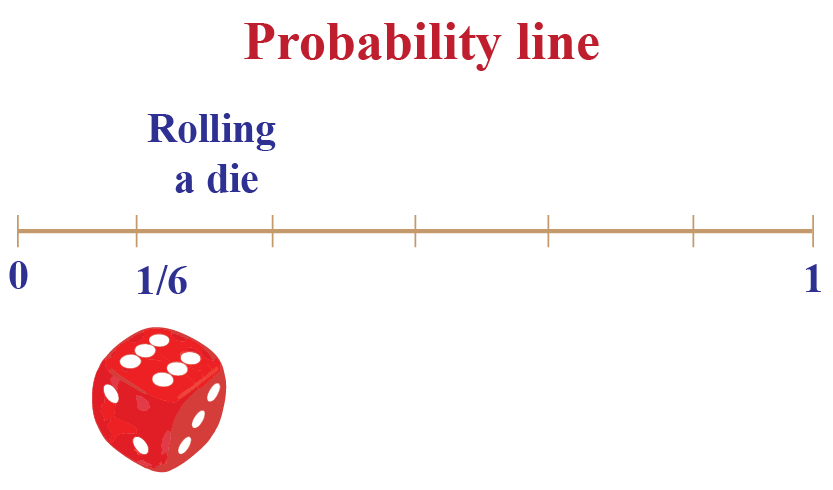## How to Determine The Probability of a Number on Dice?

### Rolling The Dice

The following table shows the number of occurrences and the relative occurrence of each outcome for a loaded die that was rolled 600 times:

 Outcome 1 2 3 4 5 6 Number of Occurrences 100 80 150 120 100 50 Relative Occurrence $$\dfrac{1}{6}$$ $$\dfrac{2}{15}$$ $$\dfrac{1}{4}$$ $$\dfrac{1}{5}$$ $$\dfrac{1}{6}$$ $$\dfrac{1}{12}$$

The relative occurrence of Face-6 is the lowest, while that of Face-3 is the highest. The sum of the probabilities is 1. This is because the sum of all the relative occurrences must be 1. The sum of occurrences

\begin{align}&=\dfrac{1}{6}+\dfrac{2}{15}+\dfrac{2}{15}+\dfrac{1}{5}+\dfrac{1}{6}+\dfrac{1}{12}\\\\ &= \dfrac{10+24+45+36+30+15}{180}\\ &=\dfrac{180}{180}\\&=1\end{align}

In the following simulation, the die is thrown 30 times at the maximum. Start the simulation and observe the frequency of the occurrences and the relative occurrence.

### Dice Probability

Suppose that our random experiment is rolling a fair die. Let us define an event E as follows:

E: A number greater than 4 shows up. Note that there are two possible outcomes in E: either 5 shows up, or 6 shows up.  Considering a large number of rolls, the relative occurrence of the outcome “5” is $$\dfrac{1}{6}$$ and the relative occurrence of the outcome “6” is also $$\dfrac{1}{6}$$. The relative occurrence of event E is
$\frac{1}{6} + \frac{1}{6} = \frac{2}{6} = \frac{1}{3}$
Thus, we can say that $$P\left( E \right) = \frac{1}{3}$$, that is the dice probability of event E occurring is $$\frac{1}{3}$$, or in other words, for a large number of rolls, event E will occur one-thirds of the time.

## Solved Examples

 Example 1

Ron throws a die. He expects an event E is defined as follows: E: A multiple of 3 shows up. What is the probability of E occurring?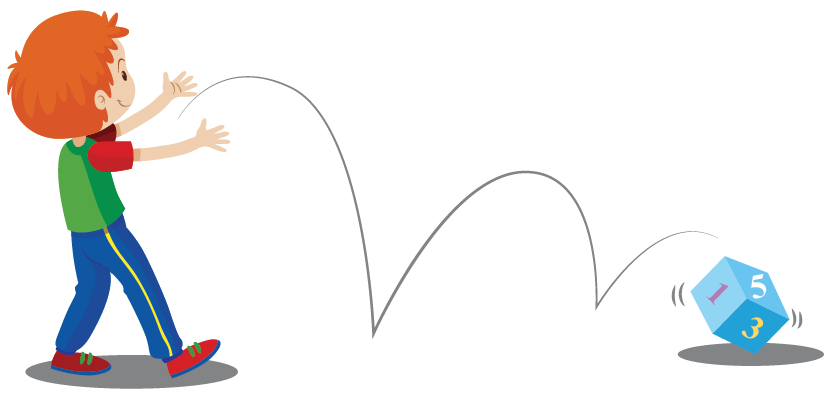Solution

The event E consists of two possible outcomes: 3 or 6. Thus, the probability of E occurring is:

\begin{align}P( E ) &= \dfrac{\text{number of favorable outcomes}}{\text{Number of total outcomes}}\\ &= \dfrac{2}{6}\\ &= \dfrac{1}{3}\end{align}

 $$\therefore$$ the probability= $$\dfrac{1}{3}$$
 Example 2

Shawn throws a die 400 times and he records the score of getting 5 as 30 times. What could be the probability of

a) getting a score of 5?

b) getting a score under 5?

Solution

a) P(getting a score of 5) \begin{align}&=\dfrac{\text{number of times getting 5}}{\text{total times}}\\\\&=\dfrac{30}{400}\\\\&= \dfrac{3}{40}\end{align}

b) P(getting a score under 5) \begin{align}&=\dfrac{\text{number of times getting under 5}}{\text{total times}}\\\\&=\dfrac{370}{400}\\\\&= \dfrac{37}{40}\end{align}

 $$\therefore$$ a) P(getting 5) $$= \dfrac{3}{40}$$ b) P(getting under 5) $$= \dfrac{37}{40}$$
 Example 3

Patrick rolls a 6-sided die. What will be the probability that he will get a prime number?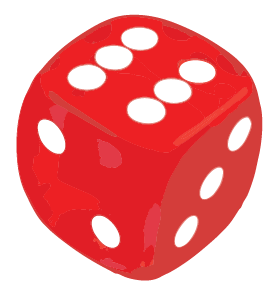Solution

The total possible outcomes in our sample space is 6

The numbers in the sample space is {1,2,3,4,5,6}

The prime numbers among these are {2,3, 5}

Probability of getting prime numbers

\begin{align}&= \dfrac{\text{favorable outcomes}}{\text{total possible outcomes}}\\ &= \dfrac{3}{ 6}\\&=\dfrac{1}{2}\end{align}

 $$\therefore$$ Probability(getting a prime number) $$=\dfrac{1}{2}$$

## Interactive Questions

Here are a few activities for you to practice. Select/Type your answer and click the "Check Answer" button to see the result.Think Tank
1. You have rolled a die 3 times in a row. You make a 3 digit number. The second digit is four times as big as the third digit, while the first digit is three less than the second digit.What is the number?## Let's Summarize

We hope you enjoyed learning about rolling a die with interactive questions. Now, you will be able to easily solve problems on probability while rolling the dice, throw the dice,  number on dice, and about a fair die.

At Cuemath, our team of math experts is dedicated to making learning fun for our favorite readers, the students!

Through an interactive and engaging learning-teaching-learning approach, the teachers explore all angles of a topic.

Be it worksheets, online classes, doubt sessions, or any other form of relation, it’s the logical thinking and smart learning approach that we, at Cuemath, believe in.

### 1. How many sides are there in a fair die?

There are 6 sides in a fair die.

### 2. How do you find the probability of rolling a die?

The probability of an event $= \dfrac{\text{favorable outcome}}{\text{total possible outcomes}}= \dfrac{1}{6}$

### 3. What is the probability of rolling 4 on a die?

$\dfrac{1}{6}$

### 4.What is the probability of rolling 2 on a 6 sided die?

$\dfrac{\text{getting 2}}{\text{total possible outcomes}}= \dfrac{1}{6}$

### 5. What is the probability of rolling an odd number?

$\dfrac{3}{6}= \dfrac{1}{2}$

### 6. What is the probability of rolling a 2 or an odd number?

\begin{align}\dfrac{1}{6}\times \dfrac{3}{6} &= \dfrac{3}{36} &=\dfrac{1}{12}\end{align}

### 7. How many even numbers are there on a die?

There are 3 even numbers on a die. They are 2,4,6

### 8. Is there a zero on dice?

No. there is no zero on a die.

### 9. Is a 3 sided die possible?

Yes, it is possible to have a 3-sided die which when rolled give us a number between 1 and 3

### 10. What are the odds of rolling 4 ones in a row?

It is the least possible way of getting 4 ones in a row as every number from 1 to 6 has an equal chance when a die is rolled.

### 11. What are the odds of rolling even numbers with 2 dice?

It is the chance of 50-50 that might occur as we have 3 odd numbers and 3 even numbers on the die and when 2 dice are rolled, we get $$\dfrac{18}{36} = \dfrac{1}{2}$$

Probability
Probability
grade 10 | Questions Set 2
Probability
Probability
grade 10 | Questions Set 1
More Important Topics
Numbers
Algebra
Geometry
Measurement
Money
Data
Trigonometry
Calculus
More Important Topics
Numbers
Algebra
Geometry
Measurement
Money
Data
Trigonometry
Calculus
Learn from the best math teachers and top your exams

• Live one on one classroom and doubt clearing
• Practice worksheets in and after class for conceptual clarity
• Personalized curriculum to keep up with school